Subsections

# Improper integrals and probability density functions

## Introduction

Improper integrals like the ones we have been considering in class have many applications, for example in thermodynamics and heat transfer. In this lab we will consider the role of improper integrals in probability, which also has many applications in science and engineering.

## Background

The first concept we need is that of a random variable. Intuitively, a random variable is used to measure an outcome whose value is not certain. For example, the number of hours that a hard disk can run before failing is a random variable because it is not the same for every drive, even if we only consider identical drives from the same production run. A few other examples of random variables that are important in science, engineering, or manufacturing are given below.
• The time it takes for a packet of information to travel from one location to another on the Internet.
• The number of miles that an automobile tire can be driven before it fails.
• The lengths of supposedly identical bolts manufactured by a particular production line.
• The speed of a particular gas molecule in a sample of a gas.

You may be more familiar with what are called discrete random variables, for example the number of heads obtained in ten tosses of a coin, which can only take a finite number of discrete values. In the case of a discrete random variable, the probability of a single outcome can be positive. For example, the probability that a single flip of a coin produces tails is 50%. The situation is very different when we consider a random variable like the number of miles a tire can be driven before failure, which can take any value from zero to something over 100,000 miles. Since there are an infinite number of possible outcomes, the probability that the tire fails at exactly some number of miles, for example 50,000 miles, is zero. However, we would expect that the probability that the tire would fail between 40,000 miles and 100,000 miles would not be zero, but would be a positive number.

A random variable that can take on a continuous range of values is called a continuous random variable. There turn out to be lots of applications of continuous random variables in science, engineering, and business, so a lot of effort has gone into devising mathematical models. These mathematical models are all based on the following definition.

Definition

We say that a random variable X is continuous if there is a function f(x), called the probability density function, such that

1.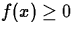, for all x
2.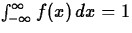3.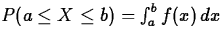where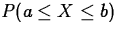represents the probability that the random variable X is greater than or equal to a but less than or equal to b.

For example, consider the following function.This function is non-negative, and also satisfies the second condition, since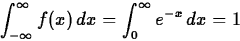which is pretty easy to show. So this could be a probability density function for a continuous random variable X.

A lot of the effort involved in modeling a random process, that is, a process whose outcome is a random variable, is in finding a suitable probability density function. Over the years, lots of different functions have been proposed and used. One thing that they all have in common, though, is that they depend on parameters. For example, the general exponential probability density function is defined as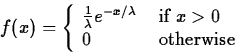where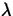is a parameter that can be adjusted to get the best fit to any particular situation. In the exercises, you will be asked to show that only positive values ofmake sense.

The process of deciding what probability density function to use and how to determine the parameters is very complicated and can involve very sophisticated mathematics. However, in the simple approach we are taking here, the problem of determining the parameter value(s) often depends on quantities that can be determined experimentally, for example by collecting data on tire failure. For our purposes, the two most important quantities are the mean,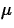and the standard deviation. The mean is defined byand the standard deviation is the square root of the variance, V, which is defined by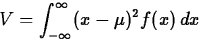In practice, the variance V is often computed as follows,which can be easily be obtained by expanding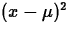and writing V as the sum of three integrals.

Probably the most important distribution is the normal distribution, widely referred to as the bell-shaped curve. The probability density function for a normal distribution with meanand standard deviationis given by the following equation.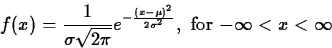This distribution has a tremendous number of applications in science, engineering, and business. The exercises provide a few simple ones.

In applications, one generally has to know in advance that the random variable you want to model has, approximately, a certain kind of distribution. How one would determine this is way beyond the scope of this course, so we won't really discuss it. On the other hand, once you know, for example, that your random variable has a normal distribution you only need the values of the mean and the standard deviation to be able to model it. The exponential distribution is even simpler, since it only has one parameter, and you only need to know the mean of your random variable to use this distribution to model it.

## Exercises

1.
Show that the probability density function given for the exponential distribution,satisfies the condition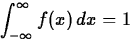as long asis a positive number. What would happen ifwas negative?
2.
Show that the mean and the standard deviation of the exponential distribution are both equal to.

3.
The amount of raw sugar that a sugar refinery can process in one day can be modeled as an esponential distribution with a mean of 4 tons. What is the probability that the refinery will process more than 4 tons in a single day?

4.
The time between accidents for all fatal accidents on scheduled American domestic passenge airplane flights for the period 1948-1961 were found to follow an exponential distribution, with a mean of approximately 44 days.
(a)
If one of those accidents occurred on July 1, find the probability that another one occurred in that same (31 day) month.
(b)
Find the variance of the time between accidents.
5.
Under average driving conditions, the life lengths of a certain brand of automobile tires follow an exponential distribution with a mean of 40,000 miles. Find the probabilities that one of these tires, bought today, would last the following numbers of miles.
(a)
Over 30,000 miles.
(b)
Over 30,000 miles, but less than 50,000 miles.

6.
The variance is defined by the following equation from the background.Show that the variance is also given by the following equation.(Hint - this might best be done with paper and pencil. Part of knowing how to use Maple is knowing when not to use it.)
7.
The median m of a random variable X is the value of the random variable such that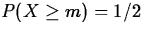. If X has an exponential distribution with mean, find the median m.

8.
The weekly amount spent for maintainence and repairs in a certain company has an approximately normal distribution with a mean of $400 and a standard deviation of$20. If \$450 is budgeted to cover repairs for next week, what is the probability that the actual costs will exceed the budgeted amount?

9.
Sick-leave time used by employees of a firm in the course of one month has approximately a normal distribution, with a mean of 200 hours and a variance of 400 hours.
(a)
Find the probability that the total sick leave for the next month will be less than 150 hours.
(b)
In planning schedules for next month, how much time should be budgeted for sick leave if that amount is to be exceeded with a probability of only 0.10?Next: About this document ... Up: No Title Previous: No Title

William W. Farr
1/19/1999